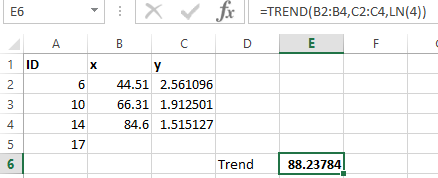Replicate Excel TREND() function in SQL Servercrompnk used Ask the Experts™
on
Hi, I'm trying to replicate the Excel TREND() function in SQL Server to get the same result. I'm using SQL Server 2016.
Below is the code to create the temporary table of values that I'm working with:

DECLARE @t TABLE
(
x FLOAT,
y FLOAT
);

INSERT INTO @t
SELECT *
FROM
(
SELECT '44.51' [x],
'12.95' [y]
UNION ALL
SELECT '66.31' [x],
'6.77' [y]
UNION ALL
SELECT '84.6' [x],
'4.55' [y]
UNION ALL
SELECT NULL [x],
NULL [y]
) T1;

In excel I can calculate the TREND using the following equation:
=TREND(B2:B4,C2:C4,LN(4))But I'd like to calculate the same result of '88.23' in SQL Server?

Thanks
Comment
Watch Question

Do more withEXPERT OFFICE® is a registered trademark of EXPERTS EXCHANGE®
IT / Software Engineering Consultant
Top Expert 2016
Commented:
First, there were some incompatibilities in what you posted.
1. The data you show in the SQL snippet does not match the values in the Excel image, which data is right?
2. I think you have the data labeled wrong, I think you have swapped the x and y headers.  At a minimum the headers are not compatible with the order you passed them to the TREND() function, they are reversed.  I assumed the headers were wrong and the TREND() usage was correct.

I don't think there is any easy way to do this SQL Server like there is in Excel, unless you have the SQL Server Analysis Services installed?  Then you may find some tools in there that can help.

If you don't, like me, then you have to write your own.  Or find someone that already has, which is the path I went for a quick test.

I did a little searching and found this page: T-SQL Starter Statistics Package for SQL Server

Pay particular note to the section titled "A stored procedure to compute the slope and intercept" since I think that is key to what you need.

(notice you can download some of the code ina ZIP file at the end of the article.)

So I took that code and added in your data (from Excel not SQL clip since I wanted to get to the answer you showed with TREND() in Excel.  I ran the code below, and got output values of:

slope = -37.868587619818
intercept = 140.734839841636

These will be used in the basic linear formula of

y = mx + b

where m = slope and b = intercept

Plugging in these results from the code below and solving for you LN(4) value of x give:

y = (-37.868587619818 * 1.386294361) + 140.734839841636

y = 88.23783036

Which was the result you seeked per your Excel example.

Okay, that's about all the time I can ghive it, hopefully this gives you a foothold up on how you might approach this, and you can do the remaining stuff to wrap and use as you need.  Good luck!

-- create and populate ##temp_xy
begin try
drop table ##temp_xy
end try
begin catch
print '##temp_xy not available to drop'
end catch

create table ##temp_xy
(
--pkID integer identity(1,1) primary key,
x float,
y float
)
go

delete from ##temp_xy

-- sample regression data
-- from --http://faculty.cas.usf.edu/mbrannick/regression/regbas.html
insert into ##temp_xy values (2.561096, 44.51)
insert into ##temp_xy values (1.912501, 66.31)
insert into ##temp_xy values (1.515127, 84.6)

-- create a fresh copy of the ##r_squared_outputs
-- to store outputs from the stored procedure to
-- compute the coefficient of determinaton and
-- correlation coefficient

begin try
drop table ##r_squared_outputs
end try
begin catch
print '##r_squared_outputs not available to drop'
end catch

create table ##r_squared_outputs
(
sum_of_y_sq_devs float
,sum_of_x_sq_devs float
,sum_of_xy_product_devs float
,correlation_coefficient float
,coefficient_of_determination float
)
go

create procedure compute_coefficient_of_determination
as
begin
set nocount on;
-- compute coefficient of determination
-- based on correlation coefficient
select
sum(y_minus_mean_sq)                                                                          sum_of_y_sq_devs,
sum(x_minus_mean_sq)                                                                          sum_of_x_sq_devs,
sum(x_y_mean_product)                                                                         sum_of_xy_product_devs,
sum(x_y_mean_product) / ( sqrt(sum(y_minus_mean_sq)) * sqrt(sum(x_minus_mean_sq)) )           correlation_coefficient,
power(sum(x_y_mean_product) / ( sqrt(sum(y_minus_mean_sq)) * sqrt(sum(x_minus_mean_sq)) ), 2) coefficient_of_determination
from
(
-- compute power deviations from mean
select
x,
y,
( x - avg_x )                 x_minus_mean,
power(( x - avg_x ), 2)       x_minus_mean_sq,
( y - avg_y )                 y_minus_mean,
power(( y - avg_y ), 2)       y_minus_mean_sq,
( x - avg_x ) * ( y - avg_y ) x_y_mean_product
from
(
-- inputs for coefficient of determination
select *
from   (select cast(x as float) x, cast(y as float)  y from ##temp_xy) source_date
cross join (select avg(cast(y as float)) avg_y from ##temp_xy) avg_y
cross join (select avg(cast(x as float)) avg_x from ##temp_xy) avg_x
) for_r_squared_inputs
) for_r_squared
return
end
go

create procedure compute_slope_intercept_correlation_coefficient
as
begin

select
slope
,(avg_y - (slope * avg_x)) intercept
,[correlation coefficient]
,[coefficient of determination]
from
(
select
(r*(s_y/s_x)) slope
,r [correlation coefficient]
,[coefficient of determination]
,(select avg(x) from ##temp_xy) avg_x
,(select avg(y) from ##temp_xy) avg_y
from
(
select
s_y
,s_x
,(select correlation_coefficient from ##r_squared_outputs) [r]
,(select coefficient_of_determination from ##r_squared_outputs) [coefficient of determination]
from
(
select
s_y
,s_x
from
(
select
stdev(x) s_x
,stdev(y) s_y
from
(
select
x
,y
from ##temp_xy
) xy
) for_s_y_and_s_x
) s_y_and_s_x
) slope
) slope_and_intercept_and_fit

return

end

go

-- Finally, invoke the compute_coefficient_of_determination
-- stored procedure followed by the compute_slope_intercept_correlation_coefficient
-- stored procedure
insert ##r_squared_outputs
exec dbo.compute_coefficient_of_determination

exec dbo.compute_slope_intercept_correlation_coefficient

»bp
Data Management Specialist

Commented:
The procedures work great, thanks.
IT / Software Engineering Consultant
Top Expert 2016

Commented:
Welcome, glad that helped.

»bp

Do more withSubmit tech questions to Ask the Experts™ at any time to receive solutions, advice, and new ideas from leading industry professionals.